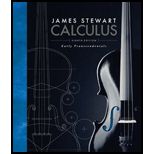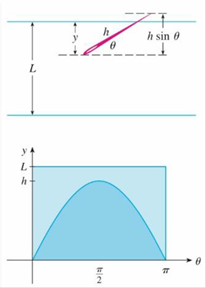# In a famous 18th-century problem. known as Buffon’s needle problem, a needle of length h is dropped onto a flat surface (for example. a table) on which parallel lines L unit s apart, L ≥ h, have been drawn. The problem is to determine the probability that the needle will come to rest intersecting one of the lines. Assume that the lines run east-west, parallel to the x -axis in a rectangular coordinate system (as in the figure). Let y be the distance from the “southern” end of the needle to the nearest line to the north. (If the needle’s southern end lies on a line, let y = 0. If the needle happens to lie east-west, let the “western” end be the “southern” end) Let θ be the angle that the needle makes with a ray extending eastward from the “southern” end. Then 0 ≤ y ≤ L and 0 ≤ θ ≤ π . Note that the needle intersects one of the lines only when y &lt; h sin θ . The total set of possibilities for the needle can be identified with the rectangular region 0 ≤ y ≤ L , 0 ≤ θ ≤ π , and the proportion of times that the needle intersects a line is the ratio area under y = h sin θ area of rectangle This ratio is the probability that the needle intersects a line. Find the probability that the needle will intersect a line if h = L. What if h = 1 2 L ?### Calculus: Early Transcendentals

8th Edition
James Stewart
Publisher: Cengage Learning
ISBN: 9781285741550### Calculus: Early Transcendentals

8th Edition
James Stewart
Publisher: Cengage Learning
ISBN: 9781285741550

#### Solutions

Chapter
Section
Chapter 8, Problem 11P
Textbook Problem

## In a famous 18th-century problem. known as Buffon’s needle problem, a needle of length h is dropped onto a flat surface (for example. a table) on which parallel lines L unit s apart, L ≥ h, have been drawn. The problem is to determine the probability that the needle will come to rest intersecting one of the lines. Assume that the lines run east-west, parallel to the x-axis in a rectangular coordinate system (as in the figure). Let y be the distance from the “southern” end of the needle to the nearest line to the north. (If the needle’s southern end lies on a line, let y = 0. If the needle happens to lie east-west, let the “western” end be the “southern” end) Let θ be the angle that the needle makes with a ray extending eastward from the “southern” end. Then 0 ≤ y ≤ L and 0 ≤ θ ≤ π. Note that the needle intersects one of the lines only when y < h sin θ. The total set of possibilities for the needle can be identified with the rectangular region 0 ≤ y ≤ L, 0 ≤ θ ≤ π, and the proportion of times that the needle intersects a line is the ratio area under  y = h sin θ area of rectangle This ratio is the probability that the needle intersects a line. Find the probability that the needle will intersect a line if h = L. What if h = 1 2 L ?Expert Solution
To determine

The probability of the needle to intersect a line h=L .

The probability of the needle to intersect a line h=12L .

### Explanation of Solution

Given information:

The needle intersects a line in the ratio of P=areaundery=hsinθareaofrectangle (1)

The limits are 0yL,0θπ

Calculation:

Find the probability of the needle to intersect a line for h=L :

Substitute L for h in Equation (1).

P=areaundery=Lsinθareaofrectangle (2)

The area under y=Lsinθ , for limits of 0θπ is expressed as,

Areaundery=Lsinθ=0πLsinθdθ

The area of rectangle is πL .

Substitute 0πLsinθdθ for areaundery=Lsinθ and πL for areaofrectangle in Equation (2).

P=0πLsinθdθπL=0πsinθdθπ=[cosθ]0ππ=cos(π)+cos(0)π

=1+1π=2π

Therefore, the probability of the needle to intersect a line h=L is 2π_

### Want to see the full answer?

Check out a sample textbook solution.See solution

### Want to see this answer and more?

Bartleby provides explanations to thousands of textbook problems written by our experts, many with advanced degrees!

See solution